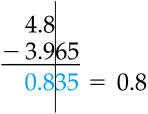# Significant FiguresThere is a general convention that the number of significant digits or figures in a measurement includes all of the decimal places that have constant value for every measurement of the parameter plus the first decimal place to the right for which the number seems to vary among measurements.

The following link discusses the general concept of significant figures and gives rules for determining how many significant figures should be included in an answer that involves the addition/subtraction of a series of numbers or the multiplication/division of a series of numbers.

Rules for Determining the Number of Significant Figures